# PCSI : Quiz d’apprentissage

## Algèbre

/10

PCSI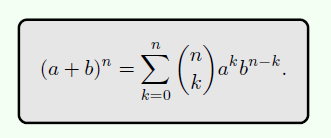Calcul algébrique

Dix questions de calcul algébrique tirées au hasard dans la base de données.

1 / 10

(x-1)(x-2) = ?

2 / 10

(1-q)(1+q+q²+q^3) =

3 / 10

(x^2)^3 =

4 / 10

P(x) = x²-5x+4 admet pour racine évidente

5 / 10

La somme des n premiers entiers est égale à

6 / 10

ln(8) =

7 / 10

La racine de 2 est environ égale à

8 / 10

Si a et b sont des réels, (a+b)^3 =

9 / 10

Comment est |x+y| par rapport à |x|+|y| ?

10 / 10

La somme pour k allant de 1 à n de ln(k+1)-ln(k) est égale à

Votre note est de

The average score is 77%

0%

PCSI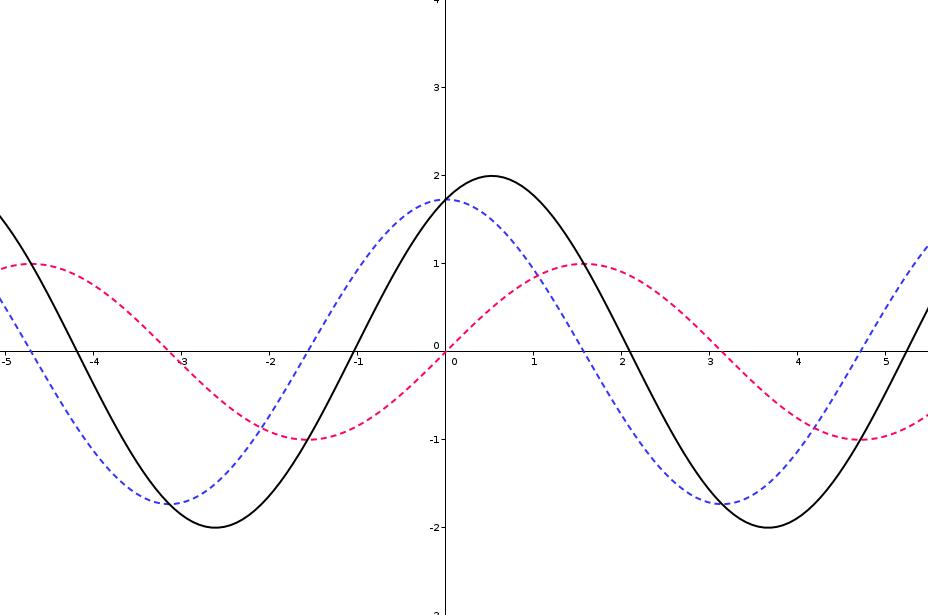Trigonométrie circulaire

Des questions autour du cercle trigonométrique : cosinus, sinus et tangente.

10 questions prises au hasard dans la base de données sur ce sujet.

1 / 10

Ceci est la représentation graphique de la fonction définie par f(x) =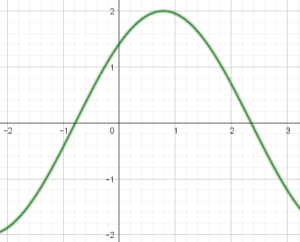2 / 10

sin(a+b)=

3 / 10

L'équation sin(x) = 1/2 d'inconnue x réelle admet

4 / 10

Si a et b sont des réels non nuls, on peut toujours écrire a*cos(t)+b*sin(t) sous la forme A*cos(t-phi)

5 / 10

Soit f la fonction définie sur R par f(x) = sin(3x). Alors une primitive F de f sur R est donnée par F(x) =

6 / 10

sin(a)sin(b)=

7 / 10

tan(pi/2) =

8 / 10

tan(pi/4) =

9 / 10

cos(a)+cos(b)=

10 / 10

sqrt(2)/2 =

Votre note est de

The average score is 61%

0%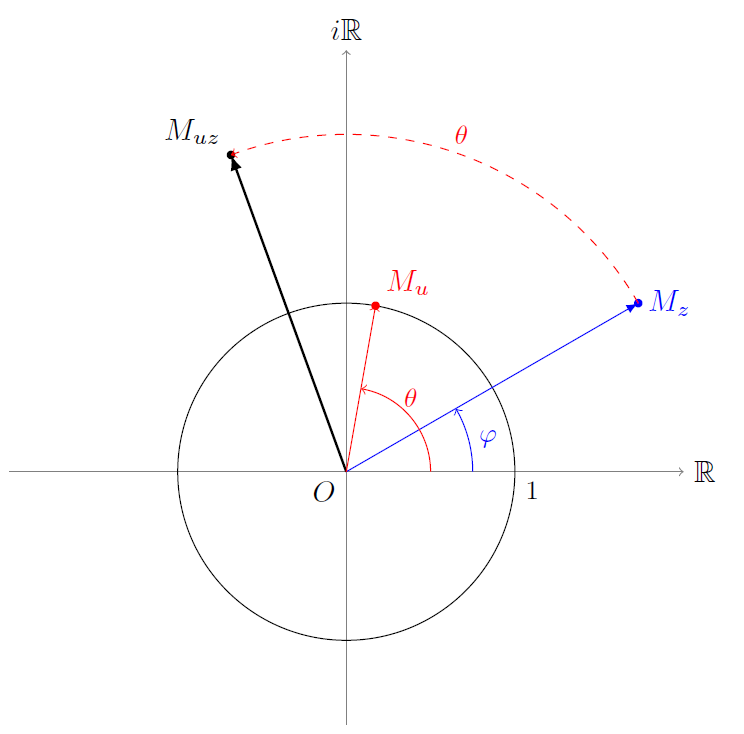Nombres complexes et trigonométrie

Des questions sur les nombres complexes et leurs relations avec la trigonométrie.

10 questions prises au hasard dans la base.

1 / 10

cos(a)+cos(b)=

2 / 10

La linéarisation de cos^3(x) est

3 / 10

Le module d'une somme est égal à la somme des modules

4 / 10

cos(a)cos(b)=

5 / 10

sqrt(2)/2 =

6 / 10

Soit z un nombre complexe non nul et u = exp(2*i*pi/3). Alors les points d'affixes z, u*z et u^2*z forment :

7 / 10

tan(pi/4) =

8 / 10

cos(2a)=

9 / 10

Le module d'un produit est

10 / 10

sin(a)cos(b)=

Votre note est de

The average score is 71%

0%

13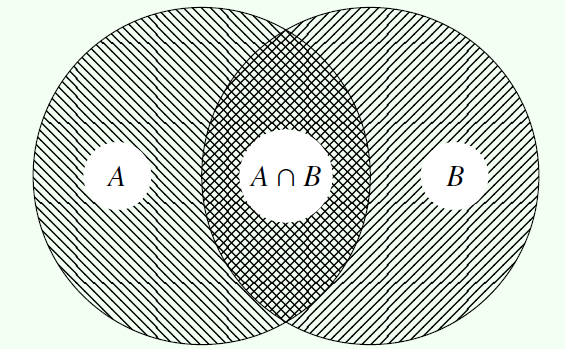Dénombrement

Des questions sur le dénombrement.

10 questions prises au hasard dans la base.

1 / 10

Combien peut-on former de matrices carrées de taille n avec des coefficients dans {-1,0,1} ?

2 / 10

Combien y a-t-il d'applications injectives de [[1,n]] vers [[1,m]], avec n<m ?

3 / 10

Combien existe-t-il de mains de cinq cartes possibles avec un jeu de 32 cartes ?

4 / 10

Le coefficient binomial "2 parmi n" est égal à

5 / 10

Combien y a-t-il de façons de cocher quatre cases distinctes dans un quadrillage à n lignes et n colonnes ?

6 / 10

Combien existe-t-il d'applications surjectives de [[1,n]] vers {0,1} ?

7 / 10

On tire simultanément p boules dans une urne contenant n boules numérotées de 1 à n. Combien y a-t-il de tirages distincts possibles ?

8 / 10

On lance successivement trois fois un dé à six faces. Combien y a-t-il de suites de résultats possibles ?

9 / 10

Combien peut on réaliser de numéros de téléphones à huit chiffres ?

10 / 10

On tire successivement et avec remise p boules dans une urne contenant n boules numérotées de 1 à n. Combien y a-t-il de tirages distincts possibles ?

Votre note est de

The average score is 72%

0%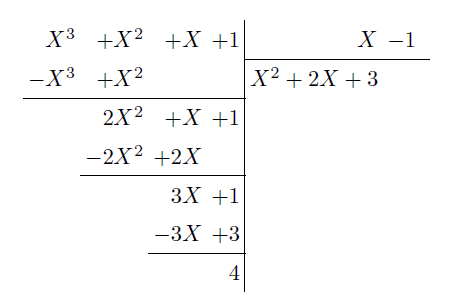Polynômes

Des questions sur les polynômes à coefficients réels ou complexes.

10 questions prises au hasard dans la base.

Si f est polynomiale et périodique, alors f est constante.

Soit P un polynôme à coefficients complexes tel que P(j) = 0. Alors

La fraction F(X) = (X^3-5X^2+8X-4)/(X^3-3X^2+2X) est irréductible

Si P est un polynôme de degré n tel que P^(k)(0) = 0 pour tout k compris entre 0 et n, alors P est nul.

Soit P = X^4+2X^2+1. Alors

Un polynôme réel de degré impair admet toujours une racine réelle.

Soit P(X) = (X-1)(X²-4X+3). Alors :

La fonction cos est polynomiale

Soit P un polynôme qui s'écrit aX²+bX+c avec (a,b,c) scalaires. Supposons que P(1) = P(-1) = P(0) = 0. Alors

Le reste de la division euclidienne de X^3-7X²+16X-9 par X-1 est nul.

Votre note est de

The average score is 63%

0%

## Analyse

PCSI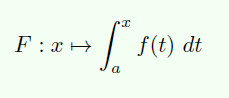Dérivées et primitives

Des calculs classiques de dérivées et de primitives.

10 questions prises au hasard dans la base.

1 / 10

Si u est dérivable, la dérivée de u^n est

2 / 10

Une primitive de tan^2(x) est

3 / 10

La dérivée de x^x est

4 / 10

La dérivée de exp(3x²+x+1) est

5 / 10

Une primitive de ln(x) est

6 / 10

Une primitive de ln(x) est

7 / 10

La dérivée de sin(2x)^5 est

8 / 10

La dérivée de ch(3x) est

9 / 10

La dérivée de ln(ln(x)) est

10 / 10

Une primitive de cos(x)sin(x) est

Votre note est de

The average score is 63%

0%

## Fondamentaux du formalisme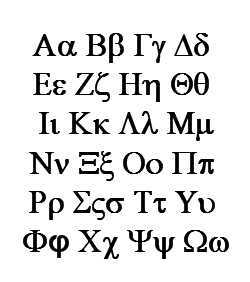Les symboles mathématiques

Alphabet grec, symboles variés, etc. Quels sont leurs noms ?

Testez vos connaissances en 10 questions prises au hasard dans la base.

1 / 10

ξ se lit

2 / 10

Θ se lit

3 / 10

∫ se lit

4 / 10

ι se lit

5 / 10

Ω se lit

6 / 10

Γ se lit

7 / 10

ψ se lit

8 / 10

λ se lit

9 / 10

Les éléments de "A×B" sont

10 / 10

"∀x" se lit

Votre note est de

The average score is 82%

0%

PCSI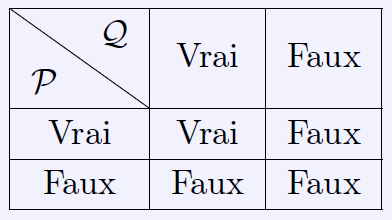Formalisme et logique

Questions autour de la logique formelle et des fondamentaux du formalisme mathématique.

1 / 10

L'assertion "Pour tout y dans [-1,1], il existe un unique x tel que cos(x)=1" est :

2 / 10

L'intersection de deux intervalles non disjoints est un intervalle

3 / 10

La contraposée de "P implique Q" est

4 / 10

Une fonction croissante et décroissante

5 / 10

Si I=[-1,2] et J = [1,3], alors I privé de J est l'ensemble :

6 / 10

La réciproque de "P implique Q" est :

7 / 10

Si f est une fonction définie sur un ensemble I, la négation de "f est strictement positive" est :

8 / 10

Soit f la fonction définie sur R par f(x) = cos(x)+1. Sélectionnez les assertions qui sont vraies.

9 / 10

"Si I et J sont des intervalles, alors la réunion de I et de J est un intervalle"

10 / 10

Si A = {1,2,3,5} et B = {2,3,4}, alors la réunion de A et de B contient :

Votre note est de

The average score is 63%

0%

## Informatique

ITC

Python - niveau 1

Dix questions simples, prises au hasard dans la base de données et portant sur les bases de la syntaxe Python. Ces questions sont pour l'essentiel accessibles dès le début du cursus en CPGE.

1 / 10

Que renvoie l'instruction suivante : (2=1) and (3>0)

2 / 10

Que renvoie l'instruction 3*'a'

3 / 10

Si L = ['a','b','c','d','e'], que renvoie L[1:3] ?

4 / 10

Après exécution de l'instruction L = [k**2 for k in range(1,10,2)], la liste L contient les valeurs :

5 / 10

Que renvoie ce bloc d'instructions ?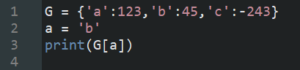6 / 10

Ce programme simule le lancer de 3 dés à six faces. S'il affiche "Gagné", cela signifie que :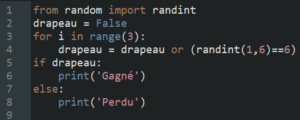7 / 10

Que renvoie l'instruction mystere(2) ?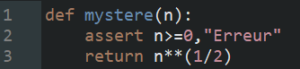8 / 10

Après exécution de ce script, que contient M ?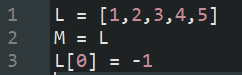9 / 10

Que contient la liste L après cette instruction ?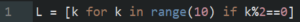10 / 10

Quel est le type de (1,2,3) ?

Votre note est de

The average score is 57%

0%